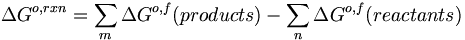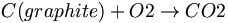# Carbon footprint to charge an iPod

## The Problem:

How much coal must you burn (and how much CO2 will you produce) to generate enough electrical energy to charge up your iPod/cellphone?

## The approximate half-cell reactions and overall reaction:

A typical lithium ion battery generates about 3.7 volts

xLi+ + xe- ⇄ Liºx(graphite) Eº = -3.05 volts

Li1-xMn2O4(s) + xLi++ xe- ⇄ LiMn2O4(s) Eº = +0.65 volts

Liºx(graphite) + Li1-xMn2O4(s) ⇄ LiMn2O4(s) Eºcell = +3.7 volts

## You can compute the maximum amount of work

Assumption #1: An average cell phone/iPod battery contains about 7 grams (1 mole of Li) Work that can be done by the battery (during discharge/energy production):

wmax = -(coulombs) · Ecell ≈

(1 mole Li) · (96,500 coulombs/mole)·(3.7 volts) ≈ -357 kJoules (in fact you won’t get this much, you might get ca. 1/2 of this amount, since you won’t use all of the available Li° and you are limited by rates of electron transfer at both anode and cathode

Energy output ≈ -178.5 kJoules

## Now the work necessary to fully charge the battery:

LiMn2O4(s) ⇄ Liºx(graphite) + Li1-xMn2O4(s) Eºcell = -3.7 volts Work that must be done to the battery (during charge/energy consumption):

wmax = -(coulombs) · Ecell ≈

(1 mole Li) · (96,500 coulombs/mole)·(3.7 volts) ≈ +357 kJoules (in fact you will have to do at least twice this much work to fully charge the battery -- same problem: available Li+ and rates of ET at both anode and cathode

Energy input ≈ +714 kJoules

## You go to standard thermodynamic tables to get the enthalpy for each of the reactants and products

Formula ΔHo,f ΔGo,f So SoP
kJ/mol kJ/mol J/K·mol J/K·mol
C(c,graphite) 0.0 0.0 5.740 8.527
C(c,diamond) 1.895 2.900 2.377 6.113
C(g) 716.682 671.257 158.096 20.838
CO2(g) (-393.509) (-394.359) 213.74 37.11
O2(g) 0.0 0.0 205.138 29.355$\Delta G^{o,rxn} = \sum_m\Delta G^{o,f}(products) - \sum_n\Delta G^{o,f}(reactants)\,\!$

Assumption

1. 2: coal = graphite$C(graphite) + O2 \rightarrow CO2\,\!$

## Then to calculate the energy per unit mass of coal

ΔHo,rxn = ΣmΔHo,f(products) - ΣnΔHo,f(reactants) = (-393.5) – ((0) + (0)) = -393.5 kJ/mole

ΔSo,rxn = ΣmSo,f(products) - ΣnΔSo,f(reactants) = (213.7) – (5.74 + 205.1) = 2.9 J/mole = 0.0029 kJ/mole

ΔGrxn ≈ ΔHo,rxn - TΔSo,rxn (assume 1000K for the combustion temperature) ≈ -393.5 kJ/mole - (1000)(.0029 kJ/mole)

ΔGrxn ≈ -396.4 kJ/mole

## Next to calculate transmission loss for power lines over a distance$C(graphite) + O2 \rightarrow CO2\,\!$

ΔGrxn ≈ -396.4 kJ/mole

By the time it gets to Tucson, and to your wall outlet, you may have lost 2/3 of that available energy (transmission losses):

Available energy to charge your battery ≈ -396.4 kJ/mole/3 = -132 kJ/mole of carbon

Energy input to charge your battery ≈ +714 kJoules

Moles of carbon (coal) needed to charge your battery ≈ +714 kJoules/132 kJ/mole = 5.4 moles of carbon

Moles of carbon (coal) needed to charge your battery ≈ +714 kJoules/132 kJ/mole = 5.4 moles of carbon

5.4 moles of carbon >> 65 grams of carbon

454 grams/lb >>> requires 0.14 lb of coal(carbon,graphite) to charge your iPod.

How much CO2 is generated? Mole CO2 = moles C = 5.4 moles of CO2

Grams of CO2 = 44 grams/mole x 5.4 moles = 238 grams of CO2 :

You generate ca. ½ pound of CO2 per charge!